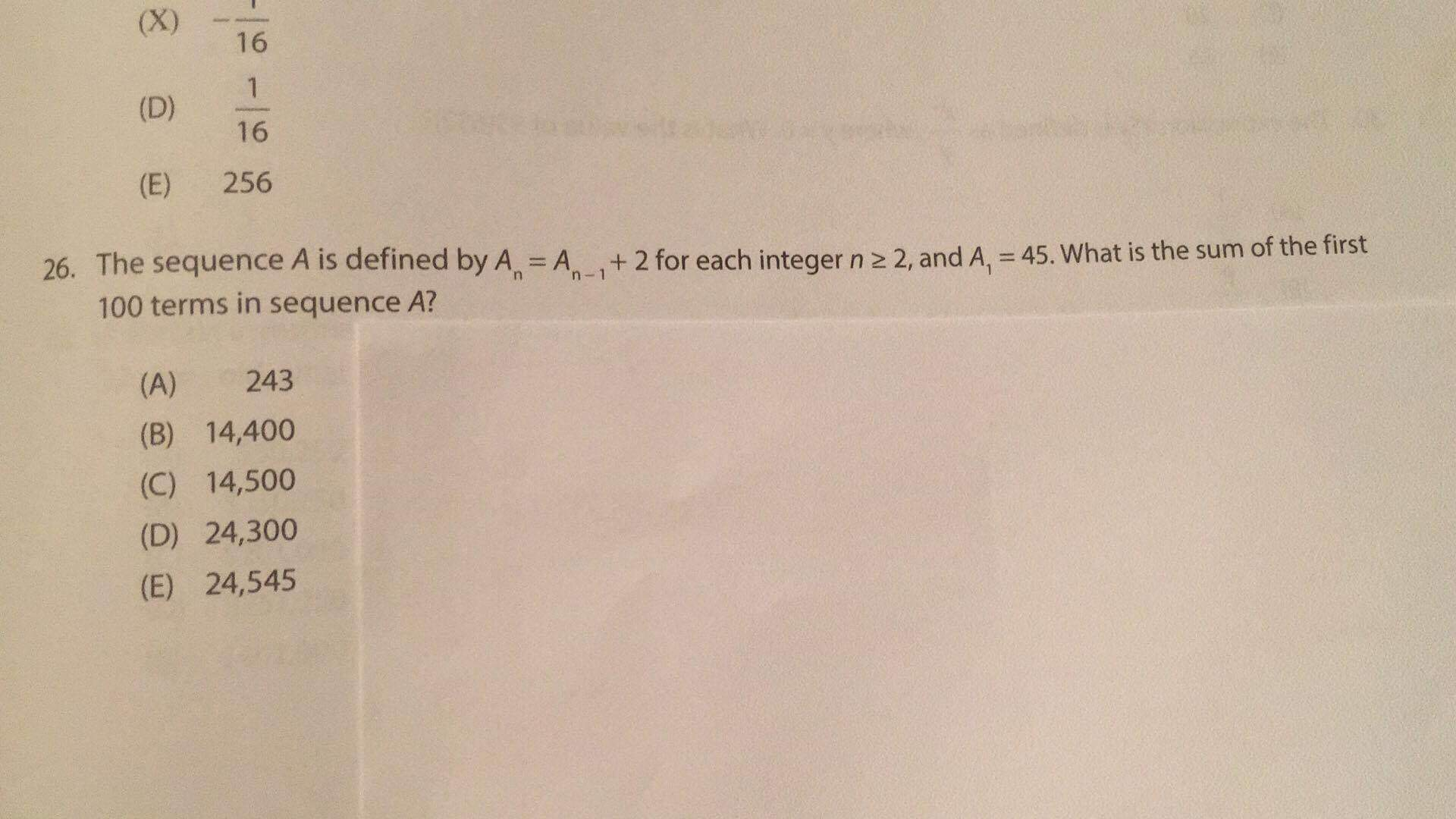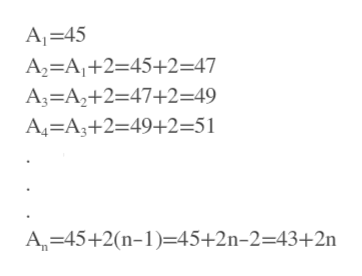(X)16(D)16(E)25626. The sequence A is defined by A100 terms in sequence A?A +2 for each integer n 2 2, and A, = 45. What is the sum of the firstn-1243(A)(B)14,400(C) 14,500(D) 24,300(E)24,545al-al-

Questionhelp_outlineImage Transcriptionclose(X) 16 (D) 16 (E) 256 26. The sequence A is defined by A 100 terms in sequence A? A +2 for each integer n 2 2, and A, = 45. What is the sum of the first n-1 243 (A) (B) 14,400 (C) 14,500 (D) 24,300 (E) 24,545 al-al- fullscreen
Step 1

Given, A_1=45 and An=A_(n-1)+2. Using n=2,3,4,.. in this ex...help_outlineImage TranscriptioncloseA 45 A2=A1+2=45+2=47 A3 A2+2=47+2=49 A4=A3+2=49+2=51 A 45+2(n-1=45+2n-2=43+2n fullscreen

Want to see the full answer?

See Solution

Want to see this answer and more?

Our solutions are written by experts, many with advanced degrees, and available 24/7

See Solution
Tagged in

Algebra